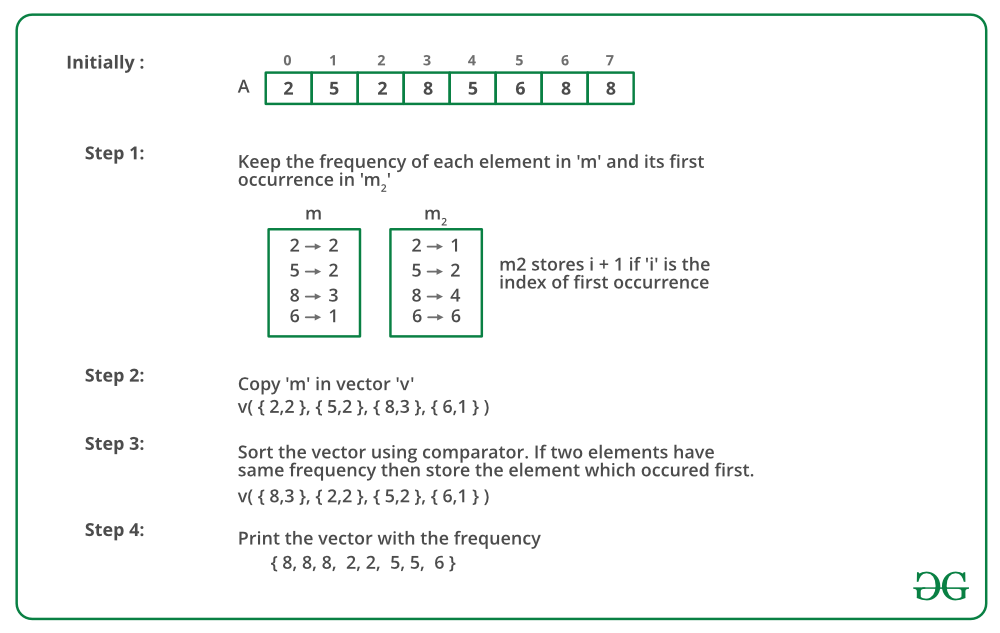# Sort elements by frequency | Set 4 (Efficient approach using hash)

• Difficulty Level : Hard
• Last Updated : 19 Jul, 2022

Print the elements of an array in the decreasing frequency if 2 numbers have the same frequency then print the one which came first.

Examples:

```Input : arr[] = {2, 5, 2, 8, 5, 6, 8, 8}
Output : arr[] = {8, 8, 8, 2, 2, 5, 5, 6}

Input : arr[] = {2, 5, 2, 6, -1, 9999999, 5, 8, 8, 8}
Output : arr[] = {8, 8, 8, 2, 2, 5, 5, 6, -1, 9999999}
```

We have discussed different approaches in below posts :
Sort elements by frequency | Set 1
Sort elements by frequency | Set 2
Sorting Array Elements By Frequency | Set 3 (Using STL)
All of the above approaches work in O(n Log n) time where n is total number of elements. In this post, a new approach is discussed that works in O(n + m Log m) time where n is total number of elements and m is total number of distinct elements.

The idea is to use hashing

1. We insert all elements and their counts into a hash. This step takes O(n) time where n is number of elements.
2. We copy the contents of hash to an array (or vector) and sort them by counts. This step takes O(m Log m) time where m is total number of distinct elements.
3. For maintaining the order of elements if the frequency is the same, we use another hash which has the key as elements of the array and value as the index. If the frequency is the same for two elements then sort elements according to the index.

The below image is a dry run of the above approach:We do not need to declare another map m2, as it does not provide the proper expected result for the problem.

instead, we need to just check for the first values of the pairs sent as parameters in the sortByVal function.

Below is the implementation of the above approach:

## C++

 `//     CPP program for the above approach ` `#include ` `using` `namespace` `std;`   `// Used for sorting by frequency. And if frequency is same,` `// then by appearance` `bool` `sortByVal(``const` `pair<``int``, ``int``>& a, ` `                      ``const` `pair<``int``, ``int``>& b)` `{`   `   ``// If frequency is same then sort by index` `   ``if` `(a.second == b.second)  ` `       ``return` `a.first < b.first;`   `   ``return` `a.second > b.second;` `}`   `// function to sort elements by frequency` `vector<``int``>sortByFreq(``int` `a[], ``int` `n)` `{`   `   ``vector<``int``>res;`   `   ``unordered_map<``int``, ``int``> m;`   `   ``vector > v;`   `   ``for` `(``int` `i = 0; i < n; ++i) {`   `       ``// Map m is used to keep track of count  ` `       ``// of elements in array` `       ``m[a[i]]++;      ` `   ``}`   `   ``// Copy map to vector` `   ``copy(m.begin(), m.end(), back_inserter(v));`   `   ``// Sort the element of array by frequency` `   ``sort(v.begin(), v.end(), sortByVal);`   `   ``for` `(``int` `i = 0; i < v.size(); ++i)  ` `      ``while``(v[i].second--)` `      ``{` `              ``res.push_back(v[i].first);` `      ``}`   `   ``return` `res;` `}`   `// Driver program` `int` `main()` `{`   `   ``int` `a[] = { 2, 5, 2, 6, -1, 9999999, 5, 8, 8, 8 };` `   ``int` `n = ``sizeof``(a) / ``sizeof``(a);` `   ``vector<``int``>res;` `   ``res = sortByFreq(a, n);`   `   ``for``(``int` `i = 0;i < res.size(); i++)` `         ``cout<

## Python3

 `# Used for sorting by frequency. And if frequency is same,` `# then by appearance` `from` `functools ``import` `cmp_to_key`   `def` `sortByVal(a,b):`   `    ``# If frequency is same then sort by index` `    ``if` `(a[``1``] ``=``=` `b[``1``]):` `        ``return` `a[``0``] ``-` `b[``0``]`   `    ``return` `b[``1``] ``-` `a[``1``]`   `# function to sort elements by frequency` `def` `sortByFreq(a, n):` `    ``res ``=` `[]` `    ``m ``=` `{}` `    ``v ``=` `[]`   `    ``for` `i ``in` `range``(n):`   `        ``# Map m is used to keep track of count` `        ``# of elements in array` `        ``if``(a[i] ``in` `m):` `            ``m[a[i]] ``=` `m[a[i]]``+``1` `        ``else``:` `            ``m[a[i]] ``=` `1`   `    ``for` `key,value ``in` `m.items():` `        ``v.append([key,value])`   `    ``# Sort the element of array by frequency` `    ``v.sort(key ``=` `cmp_to_key(sortByVal))`   `    ``for` `i ``in` `range``(``len``(v)):` `        ``while``(v[i][``1``]):` `            ``res.append(v[i][``0``])` `            ``v[i][``1``] ``-``=` `1`   `    ``return` `res`     `# Driver program`   `a ``=` `[ ``2``, ``5``, ``2``, ``6``, ``-``1``, ``9999999``, ``5``, ``8``, ``8``, ``8` `]` `n ``=` `len``(a)` `res ``=` `[]` `res ``=` `sortByFreq(a, n)`   `for` `i ``in` `range``(``len``(res)):` `    ``print``(res[i],end ``=` `" "``)`     `# This code is contributed by shinjanpatra`

## Javascript

 ``

Output

`8 8 8 2 2 5 5 -1 6 9999999 `

Time Complexity : O(n) + O(m Log m) where n is total number of elements and m is total number of distinct elements
Auxiliary Space: O(n)

This article is contributed by Aarti_Rathi and Ankur Singh and improved by Ankur Goel. If you like GeeksforGeeks and would like to contribute, you can also write an article using write.geeksforgeeks.org or mail your article to review-team@geeksforgeeks.org. See your article appearing on the GeeksforGeeks main page and help other Geeks.

My Personal Notes arrow_drop_up
Recommended Articles
Page :